Courses

# Test: Equations- 2

## 40 Questions MCQ Test Business Mathematics and Logical Reasoning & Statistics | Test: Equations- 2

Description
This mock test of Test: Equations- 2 for CA Foundation helps you for every CA Foundation entrance exam. This contains 40 Multiple Choice Questions for CA Foundation Test: Equations- 2 (mcq) to study with solutions a complete question bank. The solved questions answers in this Test: Equations- 2 quiz give you a good mix of easy questions and tough questions. CA Foundation students definitely take this Test: Equations- 2 exercise for a better result in the exam. You can find other Test: Equations- 2 extra questions, long questions & short questions for CA Foundation on EduRev as well by searching above.
QUESTION: 1

Solution:
QUESTION: 2

Solution:
QUESTION: 3

### Find the fraction which is equal to 1/2 when both its numerator and denominator are increased by 2. It is equal to 3/4 when both are incresed by 12.

Solution:
QUESTION: 4

The age of a person is twice the sum of the ages of his two sons and five years ago his age was thrice the sum of their ages. Find his present age.

Solution:

Let the age of father be x and that of his son be a,b

We have x=2(a+b) and we have been given that 5 years ago we had the following situation which is given by the equation x-5=3(a+b-10)

On solving the above equations we obtain

a+b=25 thus the age of father is 50

QUESTION: 5

A number between 10 and 100 is five times the sum of its digits. If 9 be added to it the digits are reversed find the number.

Solution:
QUESTION: 6

The wages of 8 men and 6 boys amount to Rs. 33. If 4 men earn Rs. 4.50 more than 5 boys determine the wages of each man and boy.

Solution:
QUESTION: 7

A number consisting of two digits is four times the sum of its digits and if 27 be added to it the digits are reversed. The number is :

Solution:
QUESTION: 8

Of two numbers, 1/5th of the greater is equal to 1/3rd of the smaller and their sum is 16.The numbers are:

Solution:
QUESTION: 9

Y is older than x by 7 years 15 years back X’s age was 3/4 of Y’s age. Their present ages are:

Solution:
QUESTION: 10

The sum of the digits in a three digit number is 12. if the digits are reversedthe number is increased by 495 but reversing only of ten’s and the unit digits increases the number by 36

Solution:
QUESTION: 11

Two numbers are such that twice the greater number exceeds twice the smaller one by 18 and 1/3 of the smaller and 1/5 of the greater number are together 21. The numbers are :

Solution:
QUESTION: 12

The demand and supply equations for a certain commodity are 4q + 7p = 17 and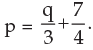respectively where p is the market price and q is the quantity then the equilibrium price and quantity are:

Solution:
QUESTION: 13

If the roots of the equation 2x2 + 8x – m3 = 0 are equal then value of m is

Solution:
QUESTION: 14

If 22x + 3 – 32. 2x + 1 = 0 then values of x are

Solution:
QUESTION: 15

Solution:

QUESTION: 16

If œ ß be the roots of the equation 2x2 – 4x – 3 = 0 the value of α2 + β2 is

Solution:
QUESTION: 17

If the sum of the roots of the quadratic equation ax2 + bx + c = 0 is equal to the sum of the squares of their reciprocals then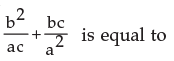Solution:
QUESTION: 18

The equation x2 –(p+4)x + 2p + 5 = 0 has equal roots the values of p will be.

Solution:
QUESTION: 19

The roots of the equation x2 + (2p–1)x + p2 = 0 are real if.

Solution:
QUESTION: 20

If x = m is one of the solutions of the equation 2x2 + 5x – m = 0 the possible values of m are

Solution:
QUESTION: 21

If p and q are the roots of x2 + x + 1 = 0 then the values of p3 + q3 becomes

Solution:

QUESTION: 22

If L + M + N = 0 and L M N are rationals the roots of the equation (M+N–L) x2 + (N+L–M)x + (L+M–N) = 0 are

Solution:
QUESTION: 23

If ∝ and ß are the roots of x2 = x+1 then value of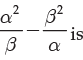Solution:
QUESTION: 24

If p ≠ q and p2 = 5p – 3 and q2 = 5q – 3 the equation having roots as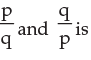Solution:
QUESTION: 25

If one rot of 5x2 + 13x + p = 0 be reciprocal of the other then the value of p is

Solution:
QUESTION: 26

A solution of the quadratic equation (a+b–2c)x2 + (2a–b–c)x + (c+a–2b) = 0 is

Solution:
QUESTION: 27

If the root of the equation x2–8x+m = 0 exceeds the other by 4 then the value of m is

Solution:
QUESTION: 28

The values of x in the equation 7(x+2p)2 + 5p2 = 35xp + 117p2 are

Solution:
QUESTION: 29

The solutions of the equation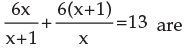Solution:
QUESTION: 30

The satisfying values of x for the equation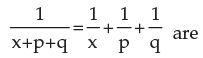Solution:
QUESTION: 31

Time taken by a train to slow down from 80 kmh-1 to 20 kmh-1 with a uniform deceleration of 2 ms-2 is

Solution:
QUESTION: 32

The values of x satisfying the equation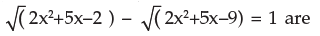Solution:
QUESTION: 33

The solution of the equation 3x2–17x + 24 = 0 are

Solution:
QUESTION: 34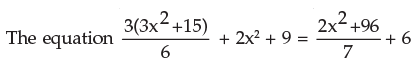has got the solution as

Solution:
QUESTION: 35

The equation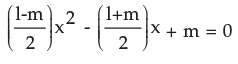has got two values of x to satisfy the equation given as

Solution:
QUESTION: 36

Te sum of two numbers is 8 and the sum of their squares is 34. Taking one number as x form an equation in x and hence find the numbers. The numbers are

Solution:
QUESTION: 37

The difference of two positive integers is 3 and the sum of their squares is 89. Taking the smaller integer as x form a quadratic equation and solve it to find the integers. The integers are.

Solution:
QUESTION: 38

Five times of a positive whole number is 3 less than twice the square of the number. The number is

Solution:
QUESTION: 39

The area of a rectangular field is 2000 sq.m and its perimeter is 180m. Form a quadratic equation by taking the length of the field as x and solve it to find the length and breadth of the field. The length and breadth are

Solution:
QUESTION: 40

Two squares have sides p cm and (p + 5) cms. The sum of their squares is 625 sq. cm. The sides of the squares are

Solution: